How To Solve Percentage Questions

What is a Percentage?

A percentage is a way of calculating how much there is of something in relation to the whole. Percentages are used very widely in both mathematics and everyday situations, and they are really useful for understanding relative amounts and making them meaningful.

Here are some common ways that percentages are used in day-to-day life:

• Calculating how well a student has performed on a test
• Working out how much VAT you need to pay on a purchase
• Calculating how much to leave as a tip in a restaurant

Percentages are usually represented by the % symbol, and there are a few basic rules you need to understand to be able to manipulate percentages: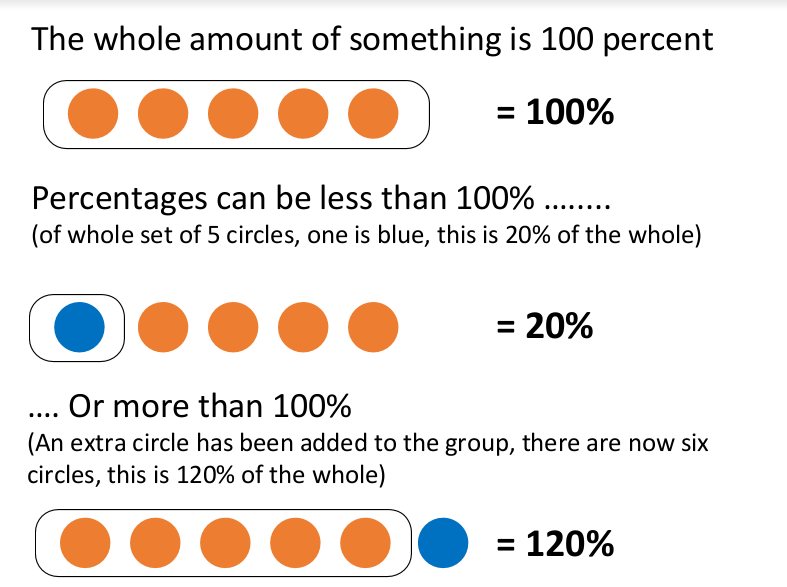Percentages are widely used in numerical reasoning tests, so it is important that you are able to understand and interpret them effectively.

Converting Decimals and Fractions to Percentage Values

When taking a numerical reasoning test, you may be required to move fluidly between questions using percentages, fractions and decimals. It is very straightforward to convert numbers between these different representations, and these are key techniques to learn.

To translate fractions into percentages, you should divide the bottom number in the fraction by the top number. This will give a decimal figure. Then multiply that decimal figure by 100, to create the percentage. Here’s an example: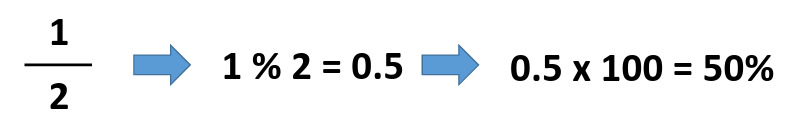To translate decimals into percentages is even easier. Simply multiply the decimal figure by 100 to calculate the percentage: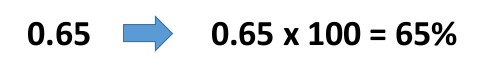Finally, to convert percentages into decimals is also very straightforward. You need to divide the percentage by 100 to calculate the decimal:How to Work Out the Percentage of a Known Value

This is a particularly useful technique, as it allows you to work out how much of a whole value a particular percentage would be. This is the formula you would need to use: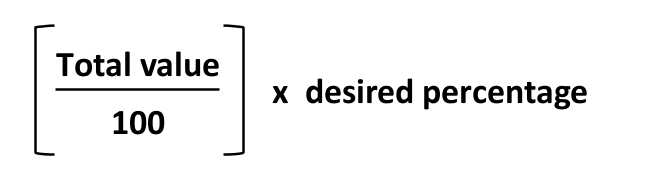This is something that people use all the time. For example, if you went out for dinner, spent £56 and wanted to leave a 10% tip, this is how you would work it out:

(£56/100) x 10 = £5.60

There are ten practice questions below. Should you need further practice afterwards, we recommend the practice packages available from JobTestPrep. These tests include percentages questions, with full explanations for all answers.

Question 1: Calculating the Percentage of a Known Value

Calculating the percentage of a known value is quite straightforward. In a numerical reasoning test, these questions tend to require you to identify/manipulate the relevant information in order to use the formula. Graphs or tables will often be used to present the information, such as the one below.

Table 1: Passenger Numbers Using Rail Services at Different Times of DayQuestion 1a. What percentage of passengers travelling between London and Brighton travelled before 12:01?

To solve this question, you need to:

• First add together all of the passengers that travelled between London and Brighton (850 + 800 + 750 + 600 = 3000)
• Then add together all of the passengers that travelled between London and Brighton before 12:01 (850 + 800 + 750 = 2400)
• Then use the formula: (2400/3000) x 100 = 80%

Question 1b. What percentage of the total passengers travelled from London to Edinburgh?

To solve this question, you need to:

• First add together all of the passengers in the table: (850 + 800 + 750 + 600 + 1100 + 2350 + 1870 + 1500 + 620 + 1400 + 980 + 790 = 13610)
• Then add together all of the passengers that travelled between London and Edinburgh (620 + 1400 + 980 + 790 = 3790)
• Then use the formula: (3790/13610) x 100 = 27.8%

Question 1c. What percentage passengers travelling between 10:31 and 12:00 were going to Birmingham?

To solve this question, you need to:

• First add together all of the passengers travelling between 10:31 and 12:00: (750 + 1870 + 980 = 3600)
• Then work out how many passengers travelled to Birmingham between 10:31 and 12:00 by looking this up in the table. The answer is 1870.
• Then use the formula: (1870/3600) x 100 = 52%

Calculating What Percentage One Number is of Another Number

This allows you to work out what percentage of the total a particular value contributes. It is easiest to explain how to do this with an example. Imagine you had 3 green apples (this is value A) and 5 red apples (this is value B). Together they give 8 apples, which is 100% of the apples you have.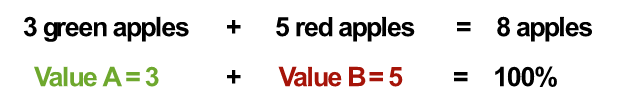To work out what percentage of the apples are green you need to use the following formula: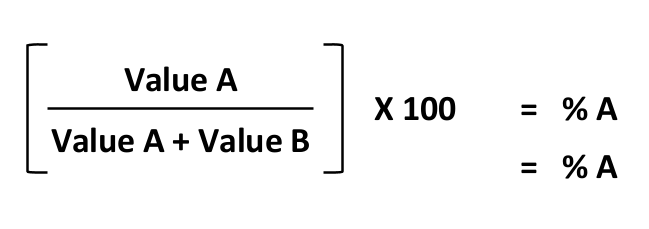The two percentages should then add up to 100%, so you can check your answers by adding them together and making sure they equal 100%.

Question 2: Calculating what percentage one number is of another

In class 11b there are 12 girls and 18 boys. What percentage of the class are girls?

To work this out you need to use the following steps:

• Work out how many children there are in the class in total: 12 + 18 = 30
• Use the formula: (12/30) x 100 = 40%

How to Work Out a Percentage Increase

Percentage increases are useful for understanding, for example, what an energy price hike means for you in financial terms, or to understand what a percentage rise in inflation equates to.

The formula you need to use to calculate this is:So, if a family originally paid £1000 per year for their energy, and their bill was increasing by £200, this is a 20% increase. The formula to calculate this is: (£200/£1000) x 100 = 20%

Using percentages like this is also useful for comparing changes to different numbers, where it can be difficult to see at a glance what the impact has been.

Question 3: Percentage Increases

Car Insurance CostsQuestion 3a: By what percentage did Tom’s car insurance increase between 2014 and 2016?

To solve this question, you need to:

• First, work out how much Tom’s car insurance increased between 2014 and 2016: £980 - £840 = £140
• Then use this formula: £140/£840 x 100 = 16.7%

So Tom’s car insurance increased by 16.7%.

Question 3b: Whose car insurance increased by the greatest percentage between 2015 and 2016?

To solve this question, you need to:

• First, work out how much each driver’s insurance increased between 2015 and 2016:

• Tom: £980 - £920 = £60
• Mohammed: £820 - £780 = £40
• Mia: £740 - £700 = £40
• Then use the formula to work out the percentage increase of each:

• Tom: £60/£920 x 100 = 6.5%
• Mohammed: £40/£780 x 100 = 5.1%
• Mia: £40/£700 x 100 = 5.7%

Tom’s car insurance therefore increased by the greatest percentage between 2015 and 2016.

Question 3c. What is the lowest percentage increase in insurance incurred by any of the drivers between 2014 and 2015?

To solve this question, you need to:

• First, work out how much each driver’s insurance increased between 2014 and 2015:

• Tom: £920 - £840 = £80
• Mohammed: £780 - £720 = £60
• Mia: £700 - £660 = £40
• Then use the formula to work out the percentage increase of each:

• Tom: £40/£840 x 100 = 9.5%
• Mohammed: £60/£720 x 100 = 8.3%
• Mia: £40/£660 x 100 = 6.1%

So the lowest percentage increase incurred by any of the drivers between 2014 and 2015 was Mia.

How to work out a percentage decrease or discount

Most people are familiar with percentage decreases or discounts, as these are used in shop sales. For example, if you want to buy a new sofa and you see that the shop is offering a 40% discount, then you know that you can achieve a substantial saving over the full price of £850.

There are two ways of calculating a percentage discount. Firstly, you can calculate the discount and then subtract this from the starting price. To do this you would use the following steps: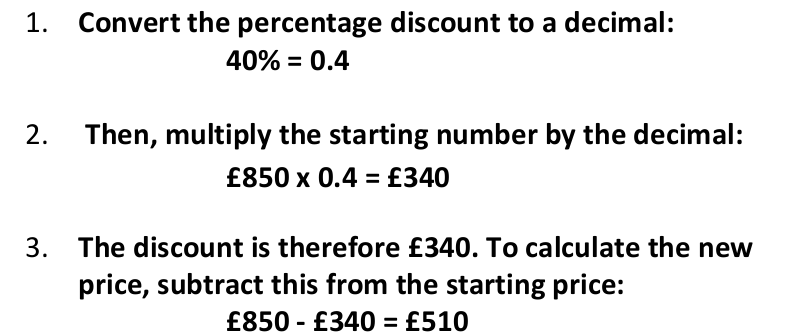An alternative methodology allows you to directly calculate what the new price would be (rather than the discount). To do this you would need to use the following steps:Suhana and Jack both want to buy a new pair of trainers. They negotiate hard and both manage to secure a discount. Suhana eventually manages to secure a 12% discount on her trainers, which originally cost £280, and Jack secures a 14% discount on his trainers with an original price of £300.

Question 4a. Who paid least for their new trainers?

To answer this question, you need to work out how much each pair of trainers cost. Using the steps above:

• Suhana: 100% - 12% = 88%, 88% = 0.88, £280 x 0.88 = £246.40
• Jack: 100% - 14% = 86%, 86% = 0.86, £300 x 0.86 = £258

Therefore, Suhana paid least for her new trainers.

Question 4b. How much was the discount that Jack managed to secure?

To answer this question, you need to work through the following steps:

• Convert the % to a decimal: 14% = 0.14
• Multiply the starting price by 0.14: £300 x 0.14 = £42

So Jack managed to secure a discount of £42.

Working backwards from a percentage

Sometimes, you may be presented with a figure that is greater than 100%. For example, if Shimmy bought a house for £150,000 in 2010, its value may increase over the years and in 5 years’ time it is worth 20% more than he paid for it.

The new value could be calculated by: Converting the percentage to a decimal: 120% = 1.2 Multiplying the starting value by 1.2: £150,000 x 1.2 = £180,000

Therefore, in 2015 his house was worth £180,000.

You can also reverse this process and work out what the starting value may have been. For example, Shimmy’s friend Elissa also bought a house in 2010. She recently had it valued and found it was now worth £230,000. This is 80% greater than what she originally paid for it. To work out the original value of the house, you need to use the following process: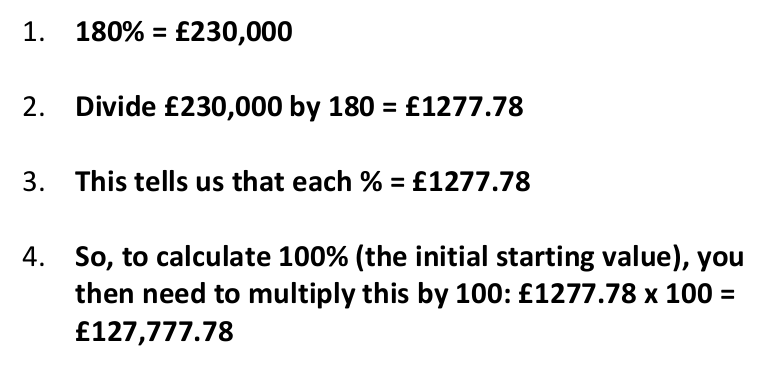Question 5: Working backwards from a percentage Grace has £3940 in her savings account. This is 25% more than she initially invested. How much was her initial investment?

To answer this, you need to go through the following steps:

• £3940 = 125%
• 3940/125 = £31.52
• £31.52 x 100 = £3152

So Grace initially invested £3152 in her savings account.

Common mistakes and things to look out for

Be careful with each of the following:

• Make sure that if you are converting decimals to percentages (or vice versa) that you get the decimal point in the right place. Often the multiple choice answers to numerical reasoning tests will include incorrect answers with exactly this error, so if you have made this mistake there may well be an answer waiting to catch you out.
• When comparing percentages make sure that you have a common baseline (otherwise the percentages will be unrelated to one another).
• One area that often catches people out is year-on-year percentage increases. For example, Freya has £10 and each year this increases by 5%. How much will she have after 3 years? Some people can be tempted to add together the 5% for the 3 years i.e. 15% and multiply the £10 by 15% giving £11.5. This is incorrect. The correct way of approaching questions like this is to remember that EACH year the initial £10 increased by 5%. So at the end of year 1, Freya would have £10 x 1.05 = £10.5. At the end of year 2, she would have £10.5 x 1.05 = 11.025, and so on. It is important to add in each of these steps to arrive at the correct answer.
• Another common error is around percentage increases. For example, the price of a loaf of bread increases by 10%. After the increase the price was £1.10, how much did the bread cost before the increase. A really common error is for people to try and solve this type of question by calculating: £1.10 x 0.9 = £0.99. This is incorrect. Remember, that £1.10 = 110%, therefore you must use this calculation: (£1.10/110) x 100 = £1.00
• Avoid using the % button on your calculator unless you are really confident in what you are doing. It might seem like a sensible short cut but it can lead to you making basic errors.

If you would like to practise more percentage-based questions, we recommend the practice packages available from JobTestPrep. These tests include numerous percentage questions, with full explanations for all answers.